# Optimal Control

Also found in: Wikipedia.

## Optimal Control

a branch of mathematics dealing with nonclassical variational problems.

Engineering deals with systems that are usually equipped with devices by which the system’s motion can be controlled. The behavior of such a system is described mathematically by equations containing parameters that characterize the position of the control devices. There naturally arises the problem of finding for the motion a control design that is in some sense the best, or optimal, one. For example, it may be desired to achieve the aim of the motion in a minimum amount of time—a problem in the calculus of variations. In contrast to classical variational problems, where the control parameters vary over a certain open, or unbounded, region, in the theory of optimal control the control parameters may assume boundary values. This fact is particularly important from an applications standpoint, since in engineering situations optimal control is often attained when a control device is at an extreme position.

The origin of optimal control dates back to the early 1950’s and is a striking example of how practical needs inevitably engender new theories. Characteristic of modern engineering and today’s highly mechanized and automated manufacturing is the desire to select the best program of action and to make the most rational use of available resources. It was precisely such practical engineering problems that stimulated the development of the theory of optimal control, which has proved to be of great mathematical importance and has enabled the solution of many problems not amenable to classical methods. The intensive development of the theory of optimal control has contributed much to the rapid solution of scientific, engineering, and economic problems.

An important result of the theory of optimal control is Pontriagin’s maximum principle, which gives a general necessary condition for optimality of control. This principle and the associated research carried out by L. S. Pontriagin and his colleagues served as a starting point for the development of the theoretical, computational, and applied aspects of the theory of optimal control. The techniques of dynamic programming, whose principles were worked out by the American scientist R. Bellman and his colleagues, have successfully been used in solving a number of problems in optimal control.

The general features of a problem in optimal control follow. Let us consider a controlled system, that is, a machine, apparatus, or process provided with control devices. By manipulating the control devices within the limits of the available control resources, we determine the motion of the system and thus control the system. For example, the process of achieving a chemical reaction can be considered a controlled system, whose control devices are the concentrations of the ingredients, the quantity of the catalyst, the temperature maintained, and the other factors influencing the course of the reaction. To know precisely how a system behaves under a control we need to know a law of motion that describes the dynamic properties of the system under consideration and determines the evolution of the state of the system for each chosen way of manipulating the control devices. The possibilities for controlling a system are limited not only by the control resources but also by the system’s not being allowed to enter states that are physically unrealizable or are impermissible from the standpoint of the specific conditions of the system’s usage. Thus, in maneuvering a ship one must take into account not only the technical capabilities of the ship but also the boundaries of the channel.

When dealing with a controlled system, one always strives to manipulate the control devices so as to move from a specific initial state to a desired end state. For example, to launch an artificial earth satellite it is necessary to calculate the engine performance of the launch vehicle that places the satellite in the desired orbit. In general, there are an infinite number of ways of controlling a system in order to achieve the goal. In this connection, there arises the problem of finding the control design enabling the attainment of the desired result in the best, or optimal, manner with respect to a specific quality criterion. Typical requirements in concrete problems are that the goal be realized in the shortest possible time, or with a minimum expenditure of fuel, or with maximum economic effect.

A typical case is a controlled system whose motion can be described by a system of ordinary differential equations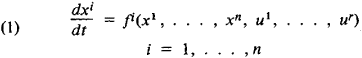where x1,…, xn are the phase coordinates characterizing the state of the controlled system at time t, and u1,…,ur are the control parameters. A control scheme for the system is a choice of control parameters as functions of time

(2) uj = uj(t) j = 1,…, r

that are permissible in view of the available capabilities for controlling the system. For example, in applied problems it is often required that at each moment of time the point (u1,…, ur) be a member of a specified closed set U, a circumstance that makes the variational problem under consideration a nonclassical one. Let the initial (x10,…, xn0) and final (x11,…,xn1) states of the system (1) be given. The control scheme (2) is said to realize the control goal if there exists a moment of time t1 > t0 such that the solution (x1(t), …. xn(t)) of the problem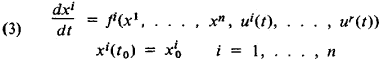satisfies the condition xi(t1)= xi1. We shall judge the quality of this control scheme by the value of the functional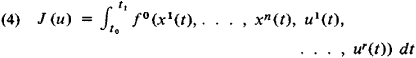where f0(x1, …, xn, u1,…,ur) is a specified function. The task of optimal control consists in finding a goal-realizing control scheme for which functional (4) assumes the minimum possible value. Thus, the mathematical theory of optimal control is a branch of mathematics that deals with the nonclassical variational problems of finding (1) extrema of functionals on the solutions of equations describing controlled systems and (2) control schemes by which such extrema can be realized.

We shall now formulate a necessary condition for optimality of control in a problem.

Pontriagin’s maximum principle. Let the vector function

(5) u = u(t) = (u1(t),…,ur(t)) t0tt1

be an optimal control scheme and the vector function

x = x(t) = (x1(t),…,xn(t)) t0tt1

be the corresponding solution of (3). Let us consider an auxiliary linear system of ordinary differential equations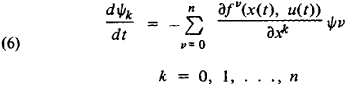and form the function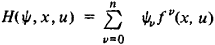which depends on the vector ψ = (ψ0, ψ1,…, ψn), as well as on x and u. Then, for the linear system (6) there exists a non-trivial solution

ψ = ψ(t) = (ψ0(t), ψ1(t),…, ψn(t)) t0tt1

such that for all points t in the interval [t0, t1] at which function (5) is continuous, the relation

max H (ψ(t), x(t), u) = ll (ψ(t), x(t), u(t)) = 0

uU

is satisfied, where ψ0(t) ≡ const ≤ 0.

The equations of motion usually reduce to the form of (1) in the case of controlled mechanical systems with a finite number of degrees of freedom. In many situations there appear other formulations of the problem of optimal control, which differ from the formulation presented above: for example, problems with fixed time, where the duration of the process is specified in advance; problems with sliding endpoints, where the initial and final states are known to belong to certain sets; and problems with phase constraints, where the solution of problem (3) must at each moment of time belong to a fixed closed set. In problems involving continuum mechanics, the quantity x characterizing the state of the controlled system is a function not only of time but also of spatial coordinates; x, for example, can describe the temperature distribution in a body at a given moment. In addition, the law of motion in such problems is a partial differential equation. It is often necessary to consider controlled systems where the independent variable assumes discrete values and the law of movement is a system of finite-difference equations. Finally, there is the theory of the optimal control of stochastic systems.

### REFERENCES

Pontriagin, L. S., V. G. Boltianskii, R. V. Gamkrelidze, and E. F. Mishchenko Matematicheskaia teoriia optimal’nykh protsessov, 2nd ed. Moscow, 1969.
Krasovskii, N. N. Teoriia upravleniia dvizheniem. Moscow, 1968.
Moiseev, N. N. Chislennye metody v teorii optimal’nykh sistem. Moscow, 1971.

N. KH. ROZOV

## Optimal Control

(Russian, ekstremal’noe regulirovanie), a method of automatic control in which the operating conditions of the controlled object are established and maintained such that the extremum value (minimum or maximum) of some criterion that characterizes the quality of the object’s operation is achieved. The criterion of quality—usually called the target function, objective function, or performance index—may be a directly measurable physical quantity, such as temperature, current, voltage, or pressure, or it may be efficiency, throughput, or some other parameter.

Optimal control is used when the behavior of the controlled object is uncertain. The necessary raw data on the object is therefore obtained first (trial actions are fed to the controlled object, the object’s reaction is studied, and those actions that change the target function in the required direction are selected); on the basis of the information obtained, working actions subsequently generated will ensure that the extremum of the quality criterion is reached (seeSEARCHING SYSTEM). Thus, optimal control performs two tasks: it finds the gradient of the target function, which determines the direction of motion to the extremum in a region of controlled coordinates in the presence of noise, perturbations, and time lags on the part of the object of optimization; and it organizes stable movement of the system in the direction of the extremum point in the shortest possible time or with a minimizing of other indicators.

The automatic device that generates control actions for the object is called an optimal controller. Optimal controllers are designed to control objects for which the dependence of the performance indicator on the control action has one extremum (a maximum or a minimum). The performance of the controller is determined by the magnitude and frequency of the trial actions, the magnitude and rate of variation in the control (working) actions, sensitivity, and other factors. Electronic, hydraulic, and pneumatic controllers for optimal control, whose structure and design features are determined by the function and range of application, are in series production in the USSR and abroad.

An optimal controller, together with the controlled object, forms an optimal control system, or optimization system. Depending on the control principle, a distinction is made between open-loop systems, which are based on the principle of control in response to a disturbance; closed-loop systems, which are based on the feedback principle; and combined systems, which use both principles simultaneously. Closed-loop optimal control systems afford high accuracy and are the type most widely used. Open-loop systems, despite many advantages (such as high speed and the absence of search motions), have limited applications, chiefly in cases where all basic disturbances acting on the controlled object can be measured. Combination-type systems exhibit the advantages of both closed-loop and open-loop systems—accuracy and speed.

The most important indicators that characterize the performance of optimal control systems depend on whether the controlled object is static or dynamic. For static objects, they include the extremum search time (the speed of the system) and the deviation of the quantity being optimized from the extremum value in the steady state (search losses). The primary indicators for dynamic objects include those listed for static objects as well as requirements regarding the nature of the transient search process (monotonicity, absence of overcontrol, and others). The selection of a specific optimal control system is usually closely connected with the specifics of the controlled object.

The first work in the field of optimal control was conducted in 1922 by M. Leblanc and T. Stein (France). The systematic study of optimal control as a new direction in the development of automatic control systems was begun in 1944 by V. V. Kazakevich (USSR, 1944); research in the field was continued in the 1950’s by C. S. Draper and Y. T. Li (USA). In the 1960’s optimal control developed into an independent field in the theory of nonlinear automatic control systems, and optimal control systems came into extensive use, for example, in the tuning of resonance circuits and automatic measuring devices, in the search for optimum parameters of models being adjusted, and in the control of chemical reactors, heaters, and flotation and crushing processes.

### REFERENCES

Krasovskii, A. A. Dinamika nepreryvnykh samonastraivaiushchikhsia sistem. Moscow, 1963.
Morosanov, I. S. Releinyeekstremal’nyesistemy. Moscow, 1964.
Kuntsevich, V. M. ¡mpul’snye samonastraivaiushchiesia i ekstremal’nye sistemy avtomaticheskogo upravleniia. Kiev, 1966.
Rastrigin, L. A. Sistemy ekstremal’nogo upravleniia. Moscow, 1974.

S. K. KOROVIN

References in periodicals archive ?
Caption: Figure 2: The cumulative effectiveness and superposed control for the optimal control and a few static controls in Example 10.
Heat exchange model between reservoirs, optimal control of temperature in the reservoir and finding of heater switch-over time are considered as well.
Consequently, instead of examining weather and climate manipulation as amorphous actions without clearly defined objectives, boundaries, and methods, we can consider operations to modify weather and climate as an optimal control problem for the ECS and its components.
The objective of this paper mainly focuses on developing a mixed discontinuous Galerkin scheme for the following control constrained optimal control problem governed by a transient convection diffusion equation:
Optimal control theory is a powerful mathematical tool which makes the decision involving complex dynamical systems.
They give a unified definition of curvature that applies to a wide class of geometric structures whose geodesics arise from optimal control problems, including Riemannian, sub-Riemannian, Finsler, and sub-Finsler spaces, but pay special attention to the sub-Riemannian (or Carnot-Caratheodory) metric spaces.
Optimal control along random periodic paths is relevant theoretically and matters to applications, e.g.
This is the only way to ensure a systematic approach to the selection of optimal control laws for propulsion electric motors during maneuvers.
To illustrate the idea we consider an optimal control problem, constrained by an evolutionary partial differential equation.
The pilot project, carried out at Tabreed's plant in Mohammed bin Zayed City, Abu Dhabi, created a prototype for an optimal control system, which was implemented following various testing phases.
The pilot project, conducted at Tabreed's plant in Mohammed bin Zayed City, Abu Dhabi, created a prototype for an optimal control system which was implemented following various testing phases.
Optimal control problems (OCPs) governed by convection diffusion partial differential equations (PDEs) arise in environmental modeling, petroleum reservoir simulation, and in many other engineering applications [12, 13, 39].

Site: Follow: Share:
Open / Close